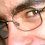## Thursday, April 12, 2012

### Dynamic Programming and Memoization

I love dynamic programming. It's a great technique to solve many optimization problems. Now if you're familiar with dynamic programming, you know that it basically consists in solving a problem with optimal sub-structure, i.e., where the optimal solution is obtained by solving one or more smaller sub-problems of the same kind, and overlapping sub-problems, i.e., when the solution is obtained by repeatedly solving the same sub-problems over and over. Dynamic programming algorithms can usually be written in a recursive fashion, i.e., with a top-down approach. For example an algorithm to compute Fibonacci numbers might call itself recursively. The algorithm can be "memoized" by storing the value of the i-th Fibonacci number right after it's computed the first time and then use the stored values when needed. Writing algorithms in a top-down fashion is usually more intuitive and easy to understand, e.g., Fn = Fn-1+Fn-2 for the Fibonacci sequence. Memoization makes sure the algorithm doesn't compute the same Fibonacci number twice, thus the running time is O(n). Note that there are smarter ways to compute Fibonacci numbers, e.g., using the recursive formulas F2n-1 = Fn2+Fn-12 and F2n = (2Fn-1+Fn)Fn, which lead to logarithmic running time.
There are also smarter ways of writing dynamic programming algorithms. In fact, memoization is great because it allows writing code in a natural way, but it leaves us with a lot of overhead due to the call stack. The solution is to write the algorithm in a bottom-up fashion instead. This means solving the simplest sub-problems first and then using them to solve the higher level sub-problems in progressive order. Going back to our Fibonacci sequence, this means computing the elements of the sequence one after the other starting from F0 = 0 and F1 = 1, F2 = F0+F1 and so on. The recursive program can be now written in iterative form, e.g., in a for loop. It's worth noting that the asymptotic running time is still O(n), but the time saved by reducing the call stack overhead will noticeably improve the constant factors, leading to a faster algorithm.
It's interesting to have an idea of this improvement. I decided to implement the cutting-rod problem with both approaches and compare the running times. I'm not going to describe the problem or the solution here, since it's really a textbook example. The figure below shows a plot of the running time for the two implementations of the rod cutting algorithm: the bottom-up one is obviously the winner with a constant factor about 3 times smaller than the top-down one (both algorithms run in O(n2)).

And here's the java code used to obtain the data in the plot. Enjoy!

`````` import java.util.Arrays;
import java.util.Random;
public class RodCutting {
public static void main(String[] args) {
// the random seed is chosen arbitrarily
Random rnd = new Random(2358761235817L);
// the class RodCutting contains the two algorithms
RodCutting cutRod = new RodCutting();
// this creates an array of rod lengths to test. 30 is the interval
// between lengths and 1500 the maximum value in the array, thus the
// array is {30,60,...,1470,1500}.
int rodLengths[] = computeRodLengths(30, 1500);
// generate 50 test cases to get consistent results
int nCases = 50;
// maximum price per rod piece
int max = 50;
// computing times in ns are stored in these two arrays
long[] computingTimeMemoized = new long[rodLengths.length];
long[] computingTimeBottomUp = new long[rodLengths.length];
for (int i = 0; i < nCases; i++) {
// create a test case
int[] p = generateTestCase(rodLengths[rodLengths.length - 1], max, rnd);
// run the algorithm for each selected rod length
for (int j = 0; j < rodLengths.length; j++) {
computingTimeMemoized[j] += testMemoized(p, rodLengths[j], cutRod);
computingTimeBottomUp[j] += testBottomUp(p, rodLengths[j], cutRod);
}
}
System.out.printf("%20s %20s %20s\n", "rod length",
"memoized time [ns]", "bottom-up time [ns]");
for (int i = 0; i < rodLengths.length; i++) {
computingTimeMemoized[i] /= nCases;
computingTimeBottomUp[i] /= nCases;
System.out.printf("%20d %20d %20d\n", rodLengths[i],
computingTimeMemoized[i], computingTimeBottomUp[i]);
}
}
public int cutRodMemoized(int[] p, int n) {
int r[] = new int[p.length + 1];
Arrays.fill(r, Integer.MIN_VALUE);
return cutRodMemoizedAux(p, n, r);
}
private int cutRodMemoizedAux(int[] p, int n, int[] r) {
if (r[n] >= 0)
return r[n];
int q = 0;
if (n != 0) {
q = Integer.MIN_VALUE;
for (int i = 1; i <= n; i++)
q = Math.max(q, p[i - 1] + cutRodMemoizedAux(p, n - i, r));
}
r[n] = q;
return q;
}
public int cutRodBottomUp(int[] p, int n) {
int[] r = new int[p.length + 1];
r = 0;
for (int j = 1; j <= n; j++) {
int q = Integer.MIN_VALUE;
for (int i = 1; i <= j; i++)
q = Math.max(q, p[i - 1] + r[j - i]);
r[j] = q;
}
return r[n];
}
private static int[] computeRodLengths(int interval, int max) {
int l = max / interval;
int[] ret = new int[l];
for (int i = 0; i < l; i++)
ret[i] = interval * (i + 1);
return ret;
}
private static long testMemoized(int[] p, int n, RodCutting cutRod) {
long start = System.nanoTime();
cutRod.cutRodMemoized(p, n);
return System.nanoTime() - start;
}
private static long testBottomUp(int[] p, int n, RodCutting cutRod) {
long start = System.nanoTime();
cutRod.cutRodBottomUp(p, n);
return System.nanoTime() - start;
}
private static int[] generateTestCase(int n, int max, Random rnd) {
int[] ret = new int[n];
for (int i = 0; i < n; i++)
ret[i] = rnd.nextInt(max);
return ret;
}
}
``````

1.2.